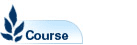# Numerical Methods and Statistics - MATH2089

Faculty: Faculty of Science

Course Outline: http://www.maths.unsw.edu.au

Campus: Kensington Campus

Units of Credit: 6

Indicative Contact Hours per Week: 6

Enrolment Requirements:

Prerequisite: MATH1231 or MATH1241 or MATH1251. Exclusions: CVEN2002, CVEN2025, CVEN2702,ECON3209, MATH2049, MATH2829, MATH2839, MATH2899, and MINE2700

Excluded: BEES2041, BIOS2041, MATH2099, MATH2801, MATH2841, MATH2859, MATH2901

Tuition Fee: See Tuition Fee Schedule

Further Information: See Class Timetable

View course information for previous years.

## Description

Numerical Methods: Numerical differentiation, integration, interpolation and curve fitting (regression analysis). Solution of linear and non-linear algebraic equations. Matrix operations, and applications to solution of systems of linear equations, elimination and tridiagonal matrix algorithms. Introduction to numerical solution of ordinary and partial differential equations.
Statistics: Exploratory data analysis. Probability and distribution theory including binomial, Poisson and normal. Large sample theory including the Central Limit Theorem. Statistical inference including estimation, confidence intervals and hypothesis testing. One-sample and two-sample tests. Linear regression. Analysis of variance. Design and analysis of experiments. Applications will be drawn from mechanical, mining, photovoltaic and chemical engineering and surveying. Matlab will be used in this course.